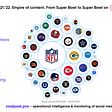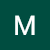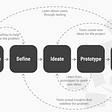# Project Introduction

We live and react with others based on our and their interactions. As nowadays, digitization takes a huge space in our daily life so most of our communication is done through digital devices. I believe that it would be hard to remember the last hand-written message you had received from your mate. Thus, most companies replace the classic way with modern technologies like web and mobile apps to be more extensive. The question is how to know the client will be interactive positively or not. The case of the project was the Starbucks rewards mobile app.

The improvement of the technology enables AI and ML to be utilized optimally. So, Udacity has been suggested this case be a capstone project in Data Scientist Nanodegree. Also, many thanks to Starbucks for publishing the dataset to develop a solution on this case.

This data set contains simulated data that mimics customer behaviour on the Starbucks rewards mobile app. Once every few days, Starbucks sends out an offer to users of the mobile app. An offer can be merely an advertisement for a drink or an actual offer such as a discount or BOGO (buy one get one free). Some users might not receive any offers during certain weeks. Also, this data set is a simplified version of the real Starbucks app because the underlying simulator only has one product whereas Starbucks actually sells dozens of products. The data is represented in three files:

• portfolio.json: containing offer ids and metadata about each offer (duration, type, difficulty, reward, duration and channels)
• profile.json: demographic data for each customer like (age, gender, income and date of became a member)
• transcript.json: records for transactions, offers received, offers viewed, and offers completed

# Problem Statement

According to the question of our case, I have developed a model that predicts users will complete the offer or not to support the marketing team for selecting the high potential clients.

The following steps which I have used to develop a model are crucial:

1- Data Exploration

2- Data Cleaning & EDA

3- Building Models

Each step has substeps to dive deeper into data for understanding all main perspectives. However, the following substeps will be shown on one dataset as an example. For more details, kindly visit the notebook click here on GitHub.

## 1- Data Exploration

This step includes displaying the set rows, how missing values, the Summary Statistics, the data types of features and the shape in each data file. Therefore, the initial patterns and the important features will be known and determine the optimal visualization for each dataset per column. Also, the high-level understanding of data and outlier detection.

Firstly, the files should be parsed into dataframe format.

`# read in the json filesportfolio = pd.read_json('data/portfolio.json', orient='records', lines=True)profile = pd.read_json('data/profile.json', orient='records', lines=True)transcript = pd.read_json('data/transcript.json', orient='records', lines=True)`

1.1 Display Data

Show the first five rows of each dataset

`portfolio.head()`

## 1.2 Data Completeness

Show how missing values in each dataset

`portfolio.isnull().sum()`

As a result, there is no missing value in this dataset.

## 1.3 Descriptive Statistics of Data

Show the Summary Statistics of each dataset

`portfolio.describe()`

According to summary statistics, `portfolio`has an average offer duration by 6.5 days

## 1.4 Data Types

Show the data types of features in each dataset

`portfolio.info()`

All columns in this dataset are in the correct format.

## 1.5 Data Shape

Display the shape of each dataset

`portfolio.shape`

## 2- Data Cleaning & EDA

To produce a useful dataset, preprocessing stage like cleaning is mandatory. Thus, the following substeps are getting the dictionary of offer and person IDs, fixing the incorrect values of columns, filling the missing values and dropping the duplicated rows. Then, visualize the dataset to find insights.

## 2.1 Fix The Incorrect Values of Columns

`# get value of channels columnchannels_data = portfolio.channels.values# cast nd array to listchannels_list = list(itertools.chain(*channels_data))# get a unique list of channels valuescleaned_channels_list = list(set(channels_list))for item in cleaned_channels_list:portfolio.insert(2,'is_'+item+'_channel',portfolio.channels.apply(lambda x: 1 if item in x else 0))# drop a columnportfolio.drop(['channels'],axis=1,inplace=True)portfolio.head()`

## 2.2 Fill The Missing Values

`# show the first 10 rows of nulls in gender columnprofile[profile.gender.isna()].head(10)`

According to above, the missing values in gender and income are related to abnormal values in the age that is 118. Thus, all those values are removed from the dataset.

## 2.3 Drop The Duplicated Rows

`len(portfolio[portfolio.duplicated('id')])`

There are no duplicated rows in portfolio dataset.

## 2.4 Data Visualization

Helper function for visualizing the bar plot

`# helper function for barplotdef barplot(data, title, rotation=0, width=8, height=4):    """    INPUT:    data - (Dataframe) a dataset    title - (Str) a title of plot    rotation - (int) a degree of X-axis ticklabels rotation    width - (float) a width of figure    height - (float) a height of figureOUTPUT:    No outputs but it will show the bar-plot    """        # sort data in descending order    data = data.sort_values(by=data.columns,ascending=False)        # set the width and height of figure    plt.figure(figsize=(width, height))        # set the title of plot    plt.title(title)        # the degree of x-ticklabels rotation    plt.xticks(rotation=rotation)        # set the data of x and y axes    plt.bar(data.index, data.iloc[:,0].values)            # Save the plot as an image    plt.savefig('fig\\'+title+'.jpg', bbox_inches = 'tight')        plt.show();`

I have used this for the following plots:

`data = portfolio[['is_email_channel','is_mobile_channel','is_social_channel','is_web_channel']].sum().to_frame()barplot(data=data, title='Count of Offer per Channel')`

According to the above graph, all offers have been sent via email channel and the least channel used is social. Thus, email is the most reabile channel for sharing offers.

`data = portfolio.groupby(by=['offer_type'])[['duration']].mean()barplot(data=data, title='Duration Average of Offer per Type')`

As shown above, the average of duration depends on offer type since the discount has the highest average which is around 8.5 days while the BOGO has 6 days.

`data = profile.groupby(by=['gender'])[['id']].count()barplot(data=data, title='Count of User per Gender')`

With regard to the bar plot, the male is the most gender of a registered person by more than 8,000 users while the female comes in the second rank by around 6,000 users. However, there were users who selected ‘Others’ by around 200.

`data = transcript.groupby(by=['offer_id'])[['is_offer_completed']].sum()barplot(data=data, title='Count of Completed Offers per Offer', width=12, height=4, rotation=75)`

According to the above graph, the number of completed offers passed 2800 at least per offer except for two offers which have no completed offers ever. However, the top 2 offers are so closed to each other by around 100 as a difference.

The other visualizations are:

`data = profile.groupby(pd.Grouper(key='became_member_on',axis=0,freq='M'))[['id']].count()plt.figure(figsize=(8,4))plt.title('Count of New User per Month')plt.savefig('fig\\Count of New User per Month.jpg', bbox_inches = 'tight')plt.plot(data.index,data.id);`

The line plot has shown that the number of new users increased yearly in general. Also, the mid of 2015 had the first jump of number from around 60 to 250 until the end of the year. The highest numbers of new users are in the second half of 2017 with 760 one time and 800 twice.

Then, I have merged those datasets into one dataset to find more insights. The following steps represent the mergation process:

• Map Person and Offer to Numeric ID
`# map person and offer IDs to their numeric versionportfolio['id'] = portfolio.id.apply(lambda x: list(offer_ids_dict.values()).index(x))profile['id'] = profile.id.apply(lambda x: list(profile_ids_dict.values()).index(x))transcript['person'] = transcript.person.apply(lambda x: list(profile_ids_dict.values()).index(x))transcript['offer_id'] = transcript.offer_id.apply(lambda x: list(offer_ids_dict.values()).index(x))`
• Unify the name of the column
`profile = profile.rename(columns={'id':'person_id'})profile.head()`
`portfolio = portfolio.rename(columns={'id':'offer_id'})portfolio.head()`
`transcript = transcript.rename(columns={'person':'person_id'})transcript.head()`

All key columns are unified now, let’s merge them together.

• Merge datasets
`transcript_profile = pd.merge(transcript,profile,on='person_id')raw_df = pd.merge(transcript_profile,portfolio,on='offer_id')raw_df.drop(['reward_y'],axis=1,inplace=True)raw_df.head()`

After that, I do visualization for the merged dataset.

`fig = plt.figure(figsize=(8,4))sns.countplot(x='offer_type',hue='gender',data=raw_df)plt.title('Count of Gender per Offer Type')plt.savefig('fig\\Count of Gender per Offer Type.jpg', bbox_inches = 'tight')plt.show();`

As a shown bar plot, the most sent type of offer is discount where we have found the highest number of each gender type on it. Moreover, the male reaches more than 10,000 which is a big number compared to other types of offer. However, the female has the same behaviour in discount and BOGO types, unlike males.

`data = raw_df.groupby(by=['is_offer_completed'])[['time']].sum()plt.figure(figsize=(12,4))plt.title('Sum of The Time Completed vs Incompleted Offers')plt.bar(list(map(str,data.index)),data.iloc[:,0].values)plt.xticks([0,1],['Incompleted','Completed'])plt.savefig('fig\\Sum of The Time Completed vs Incompleted Offers.jpg', bbox_inches = 'tight')plt.show();`

With regard to the above plot, the completed offers have consumed a double time of incompleted offers. In other words, users prefer to take amount of time to complete like reading the details and ask their friends about the offer itself if they are really interseted.

`# change the format from yyyy-mm-dd to yyyyraw_df['became_member_on'] = pd.DatetimeIndex(raw_df['became_member_on']).yearfig = plt.figure(figsize=(12,4))sns.countplot(x='became_member_on',hue='gender',data=raw_df)plt.title('Count of Gender per Year')plt.savefig('fig\\Count of Gender per Year.jpg', bbox_inches = 'tight')plt.show();`

Regrad to the shown graph, the gender of new users is most likely male in general over the past year. In 2015, the number of new feamle users has been rocketed by around 2,000 compared to 2014. In the following years, the male was the leader except in 2016 and the highest number of new male user was between 9,700 and 9,800 in 2017. The ‘other’ type of gender appeared since 2015 with a small number.

## 3. Building Models

This part of the project includes the one-hot encoding, train-test split, scaling data, developing models and comparison.

## 3.1 One-Hot encoding

`raw_df['is_male'] = raw_df.gender.apply(lambda x: 1 if x == 'M' else 0)raw_df['is_female'] = raw_df.gender.apply(lambda x: 1 if x == 'F' else 0)raw_df['is_discount'] = raw_df.offer_type.apply(lambda x: 1 if x == 'discount' else 0)raw_df['is_bogo'] = raw_df.offer_type.apply(lambda x: 1 if x == 'bogo' else 0)# because the most of new users are after 2015, we decide to map became_member_on into 1's if year after 2015 or 0'sraw_df['is_after2015'] = raw_df.became_member_on.apply(lambda x: 1 if x > 2015 else 0)# drop the used columns in one-hot encodingcleaned_raw_df = raw_df.drop(['person_id','gender','offer_type','offer_id','became_member_on'],axis=1)cleaned_raw_df.head()`

## 3.2 70–30 Train/Test Split

`# select all features for datasetX = cleaned_raw_df.iloc[:,1:]# select the target feature for datasety = cleaned_raw_df.iloc[:,0]# define the train and test datasets with the test_size 0.3 and random state 5X_train, X_test, y_train,y_test = train_test_split(X,y,test_size=0.3, random_state=5)`

## 3.3 Scaling train and test data

`# Do Scaling for datass = StandardScaler()Xs_train = ss.fit_transform(X_train)Xs_test = ss.transform(X_test)`

The data is ready for modelling after one-hot encoding and scaling to enhance the learning process of the model.

## Metrics

In the modelling part, there are many metrics that could be used to evaluate the performance of the model but I have used accuracy to compare between models.

Accuracy: measure the correct predictions over total prediction in classification. It is the most common way in model evaluation if your target is balanced. Otherwise, the other ways should be used like f-score and precision.

Accuracy = True Positives + False Positives / True Positives + False Positives + True Negatives+ False Negatives

Here’s the helper function of building model

`def build_model(model,model_name,y_test=y_test,y_train=y_train):    """    INPUT:    model - (Dataframe) a model    model_name - (Str) a name of model      OUTPUT:    accuracy_score_train - (int) an accuracy score for train dataset    accuracy_score_test - (int) an accuracy score for test dataset    """        # Fit train data into model    model.fit(Xs_train, y_train)# Predict y train and test    y_pred_train = model.predict(Xs_train)    y_pred_test = model.predict(Xs_test)cm = confusion_matrix(y_test, y_pred_test)    plt.figure(figsize=(5, 3))    sns.heatmap(cm, annot=True, fmt='d')    plt.title('Test Confusion Matrix of - '+model_name)    plt.savefig('fig\\Test Confusion Matrix of - '+model_name+'.jpg', bbox_inches = 'tight')    plt.show()return accuracy_score(y_train,y_pred_train), accuracy_score(y_test,y_pred_test)`

The models that have been developed are:

## Logistic Regression

`# instantiate Logistic Regression Modellr = LogisticRegression()# build the model and get accuracy score of train and test datasetaccuracy_score_train, accuracy_score_test = build_model(lr,"LR")# define the dictionaries of train and test scorestrain_score = {}test_score = {}train_score['LR'] = accuracy_score_traintest_score['LR'] = accuracy_score_test`

## K-Nearest Neighbors

`# instantiate KNN Modelknn = KNeighborsClassifier(n_neighbors=7)# build the model and get accuracy score of train and test datasetaccuracy_score_train, accuracy_score_test = build_model(knn,"KNN")train_score['KNN'] = accuracy_score_traintest_score['KNN'] = accuracy_score_test`

## Decision Tree

`# Instantiate DT Modeldt = DecisionTreeClassifier()# build the model and get accuracy score of train and test datasetaccuracy_score_train, accuracy_score_test = build_model(dt,"DT")train_score['DT'] = accuracy_score_traintest_score['DT'] = accuracy_score_test`

## Support Vector Machine

`# Instantiate SVC Modelsvc = SVC()# build the model and get accuracy score of train and test datasetaccuracy_score_train, accuracy_score_test = build_model(svc,"SVC")train_score['SVC'] = accuracy_score_traintest_score['SVC'] = accuracy_score_test`

## Random Forest

`# Instantiate RF Modelrf = RandomForestClassifier()# build the model and get accuracy score of train and test datasetaccuracy_score_train, accuracy_score_test = build_model(rf,"RF")train_score['RF'] = accuracy_score_traintest_score['RF'] = accuracy_score_test`

## Model Summary

`# Define dataframes has the all model with their train and test scoresscore_df = pd.DataFrame({'Models':train_score.keys(),'Train Score':train_score.values(),'Test Score':test_score.values()})score_df.sort_values('Train Score',ascending=False).\plot(kind='bar',x='Models',y=['Train Score','Test Score'],title='Train/Test Accuracy',ylabel='Accuracy');plt.savefig('fig\\Train_Test Accuracy.jpg', bbox_inches = 'tight')`

According to the above graph, we have developed a model to predict the offer will be completed or not by many models. From an accuracy perspective, all models perform well since the lowest accuracy was 84.4%, as well as `Decision Tree`, `Random Forest` and `K-Nearest Neighbors`, have an overfitting problem. In other words, those models perform well in train-set while in test-set produce more errors in test-set (unseen data). Thus, we will do tuning parameters for `Decision Tree`, try different train-test split sizes to tackle this issue and do feature selection to find the optimal set of features.

# Conclusion

Starbucks is a well-known company in the food and beverage industry specifically in coffee. To sum up amazing findings, the number of clients in the app has been rapidly in 2016 and 2017. Also, the users who spend more time on the offer, are more likely to complete the offer. The most of new or old users are male and they are interactive with discount offers mainly while the female has the same attitude against discount and BOGO types. Also, email is the most effective channel to send offers. The discount offer is featured for a long duration compared to the other types.

In the machine learning part, I have developed a model that predicts users will complete the offer or not to support the marketing team for selecting the high potential clients. The cost of finding an influenced customer will be reduced.

The next step will be to develop an interactive website to predict whether a user will complete the offer or not in order to get a benefit from the model.

--

--

--

## More from Mohammed Alali

Data Scientist | Data Analyst | Software Engineer

Love podcasts or audiobooks? Learn on the go with our new app.

## Unsupervised Machine Learning — KMeans Clustering of São Paulo Subway Stations using Foursquare…## A Guide to The Most Common FAQs While Considering a Career in Data Analytics## NFL 2021–2022: Content Empire.## 9 insights about Seattle’s Airbnb## Basic Concepts needed for Data Science work — Part 1## From Amateur to Data Scientist — 3 Months, is it enough?## Mohammed Alali

Data Scientist | Data Analyst | Software Engineer

## Classical Machine Learning and Personas## How I Applied Design Thinking to Learn Data Science